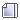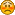###Author Topic: weighted probability  (Read 8269 times)

#### czerny

• Guest##### weighted probability
« on: January 03, 2015, 04:46:36 pm »
This is another little programming challenge!

Say, you have an array[0..MAXJOBS] of jobs (MAXJOBS 50000).
You like to select a random job from this set with the probability 1/(MAXJOBS+1).
After the job is selected, it will be executed.
The result from this execution could be TRUE or FALSE, meaning: the job is executed ok or not.
If the result is TRUE
the probability to get selected the next time should decrease a little
else
the probability to get selected the next time should increase a little

and so on.

How can this scenario be coded in C?

#### czerny

• Guest##### Re: weighted probability
« Reply #1 on: January 03, 2015, 05:39:23 pm »
This is a first solution.

It has two drawbacks:

1.) The probabilitiy is increased, when the job is not executed ok. But it is not decreased in the other case.
2.) I have to add all (~ NJOBS/2) the numerators to select the job.

Code: [Select]
`#include <stdio.h>#include <stdlib.h>#include <time.h>typedef int JOB;// random value [0;n[int uniform(int n){ int limit; int res = -1; if (n) { limit = RAND_MAX - (RAND_MAX - n + 1)%n; do res = rand(); while (res > limit); res %= n; } return res;}#define NJOBS 10int main(int argc, char **argv){ int ok, s, i, n, error=0; JOB j[NJOBS]; srand(time(NULL)); // prob = 1/(NJOBS-1), store the numerator for (i=0; i<NJOBS; i++) j[i]=1; do { // select a job n = uniform(NJOBS + error); s = 0; for (i=0; i<NJOBS; i++) { s += j[i]; if (s>n) break; }  // execute job ok = uniform(2); if (!ok) { j[i]++; error++; } printf("job[%d] ", i); } while (error<100); return 0;}`

#### dizergin

• Guest##### Re: weighted probability
« Reply #2 on: January 03, 2015, 08:34:21 pm »
To make "this scenario be coded in C"  one must have clear understanding of the model...
First of all What is a JOB?
What is the sense of the term "probability" that you used by? Is it property of a job or it has another origin...
and so on...

#### czerny

• Guest##### Re: weighted probability
« Reply #3 on: January 03, 2015, 10:25:31 pm »
First of all What is a JOB?
A job is a black box you can execute and returns a boolean value randomly.
What is the sense of the term "probability" that you used by? Is it property of a job or it has another origin...
Yes, see it as a property of each job. At the beginning, all probabilities are equal and add to 1. Later the probabilities increase/decrease for executed jobs.
See my example!

#### dizergin

• Guest##### Re: weighted probability
« Reply #4 on: January 03, 2015, 11:35:49 pm »
Yes, see it as a property of each job. At the beginning, all probabilities are equal and add to 1. Later the probabilities increase/decrease for executed jobs.
See my example!
OK,  let us  summarize it :
A job can be defined  by next structure

Code: [Select]
`typedef struct {int jobId    //job's identificatordouble P  // a float- point number within  [0,1] interval} defjob;`yet we got whole bunch of jobs:
Code: [Select]
`static defjob  jobs;`
uniformly distributed at the starting point. One can  describe it with function:
Code: [Select]
`void initializejobs ( void); // It set initial jobId and p for  each job`
The Example (with 5 jobs):
{{1,0.2},{2,0.2},{3,0.2},{4,0.2},{5,0.2}}
Let us go on...
to follow your plan one has to peek up  and execute a job from jobs with probability p...
One can  describe it with function:
Code: [Select]
`defjob  pickupjob ( double p); // It returns a random  job from subset jobs having p probability`let us look on the  example...
as we got uniform distribution at start we can do it by calling
pickupjob (0.2);
The next step depends on result of the execution choosen job
but we MUST RECALCULATE the probabilities for EVERY job...
One can  achieve it with function:
Code: [Select]
`void  normalizejobs ( defjob ajob;  double step ); // It is normalize the probability                                                                             //distribution in the such way that the sum of the p over each job = 1                                                                            // for the simplisity sake  let us regard  step as  the percent on wich we must increase(decrease) ajob's probability (depending on result of the execution)`Let us proceed with the example
let calling   pickupjob (0.2); returns  a job  with id=3
and   executing of the  job we get positive result:
in  our example  calling to
normalizejobs(ajob, 1);
gives us following :
{{1,0.1975},{2,0.1975},{3,0.21},{4,0.1975},{5,0.1975}}
if the result of the ajob execution is negative
we will get a call to
normalizejobs(ajob, -1);
resulting in
{{1,0.2025},{2,0.2025},{3,0.19},{4,0.2025},{5,0.2025}}
is it CORRECT?
« Last Edit: January 04, 2015, 12:21:53 am by dizergin »

#### czerny

• Guest##### Re: weighted probability
« Reply #5 on: January 04, 2015, 12:19:43 am »
...
and   executing the  job we get positive result:
in  the our example  next calling to
normalizejobs(ajob, 1);
gives following :
{{1,0.1975},{2,0.1975},{3,0.21},{4,0.1975},{5,0.1975}}
if the result of the ajob execution is negative
we got
normalizejobs(ajob, -1);
resulting in
{{1,0.2025},{2,0.2025},{3,0.19},{4,0.2025},{5,0.2025}}
is it CORRECT?
If the job executes ok, its probability should decrease, not increase.

#### dizergin

• Guest##### Re: weighted probability
« Reply #6 on: January 04, 2015, 12:30:45 am »
If the job executes ok, its probability should decrease, not increase.ok let us change the sign of the step value  to achieve the desired result...
Is the next call to the pickupjob  routine must refer to the subset of the jobs (with jobs that have  p - probability ) or ALL members of the  jobs array?
« Last Edit: January 04, 2015, 12:34:40 am by dizergin »

#### cfmspavia

• Guest##### Re: weighted probability
« Reply #7 on: January 04, 2015, 01:13:16 am »

Create a struct to hold the JOB:
Code: [Select]
`typedef struct {int jobId;   double JobProb;  // The probability of a job running} JOB;//Then your array:JOB MyJobs[NUMBERJOBS];`
and a variable to hold the sum of probabilities:
Code: [Select]
`double TotalJobProb;`Initialize your JOB structure array with values, where JobProb can be any number, for example 1.0

Now a function to calculate TotalJopProc:
Code: [Select]
`double CalcTotalJobProb(void) // yes, this function is needed...{double Total = 0.0;for (int i = 0; i < NUMBERJOBS; i++)Total += MyJobs.JobProb[i];return Total;}`When you need to select a Job, generate a random number between 0 and Total.

Let's call it
Code: [Select]
`double RandomNum;`Create a function to select the job
Code: [Select]
`int SelectJob(void){int i = 0;double ParcTotal = 0.0;do{ParcTotal += MyJobs.JobProb[i];i++;}while (PartTocal < RandomNum);return i-1; // this is the index of the selected array element}`

This way you only have to increase and decrease the individual JobProb values of the process you need to change probability and calcutate a new TotalProb value. No need to update all other jobs.

Sorry for the horrible formatting, but cannot figure out how to insert code blocks.

Edit: Code blocks now inserted  thanks to dizergin« Last Edit: January 04, 2015, 10:52:12 pm by cfmspavia »

#### dizergin

• Guest##### Re: weighted probability
« Reply #8 on: January 04, 2015, 01:25:37 am »
1. the forum' s engine uses BB-tags mark up   (in the square brackets)
2. At the point It is not quite clear the whole model (i see some inconsistencies)- so  (in my opinion ) let us do not be hasting with the any "solution" - for it could be appeared wrong one ( IN PRINCIPLE)....
« Last Edit: January 04, 2015, 02:23:34 am by dizergin »

#### cfmspavia

• Guest##### Re: weighted probability
« Reply #9 on: January 04, 2015, 10:54:53 pm »
Sorry about yesterday's mess with formatting and the horrible explanation. Please disregard and have a look at the attached project. Thanks.

#### cfmspavia

• Guest##### Re: weighted probability
« Reply #10 on: January 06, 2015, 12:05:59 am »
A new version of yesterday's demo program, a little more optimized and with some bug corrections.

#### czerny

• Guest##### Re: weighted probability
« Reply #11 on: January 06, 2015, 06:37:06 am »
This way you only have to increase and decrease the individual JobProb values of the process you need to change probability and calcutate a new TotalProb value. No need to update all other jobs.
You can not decrease a value of 1, so you have to start with some numbers != 1. What would be the best starting value? While increasing a value, how can you be sure that your sum will not get greater than INT_MAX?

#### cfmspavia

• Guest##### Re: weighted probability
« Reply #12 on: January 06, 2015, 11:26:52 am »
The best value for the base probability should be equal to the number of cycles that it takes for the job to get back to base (what I called TRANSITERATONSRUN), after a successful run. In this case 5 should be ok.

With this simple code you can never be sure not to reach the INT_MAX limit of the sum, but statistically unlikely. Theoretically, some job could be repeatedly selected, and keep failing on run,  and its probability could start growing and growing, affecting the sum, but if the pseudo random generator is half decent that should not happen.
The real limiting factor here is the rand() function that is only capable of numbers between 0 and 2^15. This will seriously limit the number of jobs, in an imprevisible way, as we can never be sure how much their probability on fail will increase.

I believe Pelle's C does not implement the rand_s() function that goes up to UINT_MAX. At least I could not find it.

p.s. I just realized that I implemented the function GenerateRandom() returning a long. This, of course, is just plain dumb.« Last Edit: January 06, 2015, 11:30:14 am by cfmspavia »

#### czerny

• Guest##### Re: weighted probability
« Reply #13 on: January 06, 2015, 01:29:50 pm »
The real limiting factor here is the rand() function that is only capable of numbers between 0 and 2^15. This will seriously limit the number of jobs, in an imprevisible way, as we can never be sure how much their probability on fail will increase.
This is easy to fix. But I do not like algorithmes who count on the small (~0) chance to overrun the lower or the upper limits.

#### cfmspavia

• Guest##### Re: weighted probability
« Reply #14 on: January 06, 2015, 03:37:03 pm »
Quote
But I do not like algorithmes who count on the small (~0) chance to overrun the lower or the upper limits.
That's a very wise option.